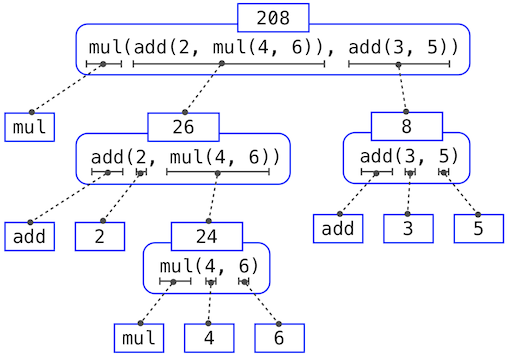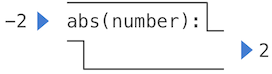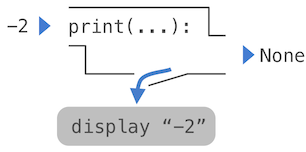# 1.2 编程元素

• 基本的表达式和语句，它们由语言提供，表示最简单的构建代码块。
• 组合的手段，复杂的元素由简单的元素通过它来构建，以及
• 抽象的手段，复杂的元素可以通过它来命名，以及作为整体来操作。

## 1.2.1 表达式

``````>>> 42
42
``````

``````>>> -1 - -1
0
>>> 1/2 + 1/4 + 1/8 + 1/16 + 1/32 + 1/64 + 1/128
0.9921875
``````

## 1.2.2 调用表达式

``````>>> max(7.5, 9.5)
9.5
``````

``````>>> pow(100, 2)
10000
>>> pow(2, 100)
1267650600228229401496703205376
``````

``````>>> max(1, -2, 3, -4)
3
``````

``````>>> max(min(1, -2), min(pow(3, 5), -4))
-2
``````

（理论上）这种嵌套没有任何限制，并且 Python 解释器可以解释任何复杂的表达式。然而，人们可能会被多级嵌套搞晕。你作为程序员的一个重要作用就是构造你自己、你的同伴以及其它在未来可能会阅读你代码的人可以解释的表达式。

## 1.2.3 导入库函数

Python 定义了大量的函数，包括上一节提到的运算符函数，但是通常不能使用它们的名字，这样做是为了避免混乱。反之，它将已知的函数和其它东西组织在模块中，这些模块组成了 Python 库。需要导入它们来使用这些元素。例如，`math`模块提供了大量的常用数学函数：

``````>>> from math import sqrt, exp
>>> sqrt(256)
16.0
>>> exp(1)
2.718281828459045
``````

`operator`模块提供了中缀运算符对应的函数：

``````>>> from operator import add, sub, mul
42
16
``````

`import`语句标明了模块名称（例如`operator``math`），之后列出被导入模块的具名属性（例如`sqrt``exp`）。

Python 3 库文档列出了定义在每个模块中的函数，例如数学模块。然而，这个文档为了解整个语言的开发者编写。到现在为止，你可能发现使用函数做实验会比阅读文档告诉你更多它的行为。当你更熟悉 Python 语言和词汇时，这个文档就变成了一份有价值的参考来源。

## 1.2.4 名称和环境

``````>>> radius = 10
10
20
``````

``````>>> from math import pi
>>> pi * 71 / 223
1.0002380197528042
``````

``````>>> area, circumference = pi * radius * radius, 2 * pi * radius
>>> area
314.1592653589793
>>> circumference
62.83185307179586
``````

`=`符号在 Python（以及许多其它语言）中叫做赋值运算符。赋值是 Python 中的最简单的抽象手段，因为它使我们可以使用最简单的名称来引用复合操作的结果，例如上面计算的`area`。这样，复杂的程序可以由复杂性递增的计算对象一步一步构建，

``````>>> max
<built-in function max>
``````

``````>>> f = max
>>> f
<built-in function max>
>>> f(3, 4)
4
``````

``````>>> f = 2
>>> f
2
``````

## 1.2.5 嵌套表达式的求解

• 求出运算符和操作数子表达式，之后
• 在值为操作数子表达式的参数上调用值为运算符子表达式的函数。

``````>>> mul(add(2, mul(4, 6)), add(3, 5))
208
``````• 数字求值为它标明的数值，
• 名称求值为当前环境中这个名称所关联的值

``````>>> add(x, 1)
``````

``````>>> x = 3
``````

## 1.2.6 函数图解

``````>>> abs(-2)
2
```````abs`是纯函数。纯函数具有一个特性，调用它们时除了返回一个值之外没有其它效果。

``````>>> print(-2)
-2
>>> print(1, 2, 3)
1 2 3
````````````>>> print(print(1), print(2))
1
2
None None
``````

``````>>> two = print(2)
2
>>> print(two)
None
``````